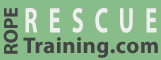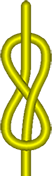# Figure 8Figure 8 knots are undeniably the most common rescue knots. They can create connection points (figure 8 on a bight), be tied directly to a harness (figure 8 follow through), and can join the ends of two ropes (figure 8 bend). They are strong, easy to recognize when tied correctly (as well as when tied incorrectly), and are relatively easy to untie after being loaded.

The knot shown here is called a "figure of 8 skeleton," because it is a bare bones figure 8 knot. The figure 8 skeleton is the starting point for the figure 8 follow through and figure 8 bend.

vRigger costs less than a rope.